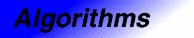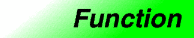# sortCategory: algorithms Component type: function

## Prototype

`Sort` is an overloaded name; there are actually two `sort` functions.

```template <class RandomAccessIterator>
void sort(RandomAccessIterator first, RandomAccessIterator last);

template <class RandomAccessIterator, class StrictWeakOrdering>
void sort(RandomAccessIterator first, RandomAccessIterator last,
StrictWeakOrdering comp);
```

## Description

`Sort` sorts the elements in `[first, last)` into ascending order, meaning that if `i` and `j` are any two valid iterators in `[first, last)` such that `i` precedes `j`, then `*j` is not less than `*i`. Note: `sort` is not guaranteed to be stable. That is, suppose that `*i` and `*j` are equivalent: neither one is less than the other. It is not guaranteed that the relative order of these two elements will be preserved by `sort`. 

The two versions of `sort` differ in how they define whether one element is less than another. The first version compares objects using `operator<`, and the second compares objects using a functors `comp`.

## Definition

Defined in the standard header algorithm, and in the nonstandard backward-compatibility header algo.h.

## Requirements on types

For the first version, the one that takes two arguments:

• `RandomAccessIterator` is a model of RandomAccessIterator.
• `RandomAccessIterator` is mutable.
• `RandomAccessIterator`'s value type is LessThanComparable.
• The ordering relation on `RandomAccessIterator`'s value type is a strict weak ordering, as defined in the LessThanComparable requirements.

For the second version, the one that takes three arguments:

• `RandomAccessIterator` is a model of RandomAccessIterator.
• `RandomAccessIterator` is mutable.
• `StrictWeakOrdering` is a model of StrictWeakOrdering.
• `RandomAccessIterator`'s value type is convertible to `StrictWeakOrdering`'s argument type.

## Preconditions

• `[first, last)` is a valid range.

## Complexity

`O(N log(N))` comparisons (both average and worst-case), where `N` is `last - first`. 

## Example

```int A[] = {1, 4, 2, 8, 5, 7};
const int N = sizeof(A) / sizeof(int);
sort(A, A + N);
copy(A, A + N, ostream_iterator<int>(cout, " "));
// The output is " 1 2 4 5 7 8".
```